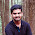### Parser for IF-THEN-ELSE Statements - YACC Program - Compiler Design

Program:

(Lex Program: ift.l)
alpha [A-Za-z]
digit [0-9]
%%
[ \t\n]
if    return IF;
then    return THEN;
else    return ELSE;
{digit}+    return NUM;
{alpha}({alpha}|{digit})*    return ID;
"<="    return LE;
">="    return GE;
"=="    return EQ;
"!="    return NE;
"||"    return OR;
"&&"    return AND;
.    return yytext;
%%

(Yacc Program: ift.y)

%{
#include <stdio.h>
#include <stdlib.h>
%}
%token ID NUM IF THEN LE GE EQ NE OR AND ELSE
%right '='
%left AND OR
%left '<' '>' LE GE EQ NE
%left '+''-'
%left '*''/'
%right UMINUS
%left '!'
%%

S      : ST {printf("Input accepted.\n");exit(0);};
ST    : IF '(' E2 ')' THEN ST1';' ELSE ST1';'
| IF '(' E2 ')' THEN ST1';'
;
ST1  : ST
| E
;
E    : ID'='E
| E'+'E
| E'-'E
| E'*'E
| E'/'E
| E'<'E
| E'>'E
| E LE E
| E GE E
| E EQ E
| E NE E
| E OR E
| E AND E
| ID
| NUM
;
E2  : E'<'E
| E'>'E
| E LE E
| E GE E
| E EQ E
| E NE E
| E OR E
| E AND E
| ID
| NUM
;

%%

#include "lex.yy.c"

main()
{
printf("Enter the exp: ");
yyparse();
}

Output:

students@cselab-desktop:~\$ lex ift.lex
students@cselab-desktop:~\$ yacc ift.y
students@cselab-desktop:~\$ gcc y.tab.c -ll -ly
students@cselab-desktop:~\$ ./a.out
Enter the exp: if(a==1)  then b=1; else b=2;
Input accepted.
students@cselab-desktop:~\$

1.Thank you very much i was looking for this

2.3.Thanks.. Nice one. But sometimes "#include "lex.yy.c" causes declaration error.

4.so dont use lex.yy.c while running the program on terminal
use : cc y.tab.c -ll -lm

5.Thanks. This was very useful

6.This comment has been removed by the author.

7.The parser is not working for if ( a == b) then b=1;
its not accepting the input